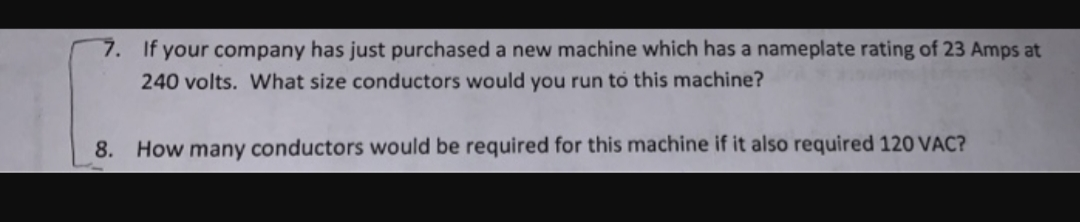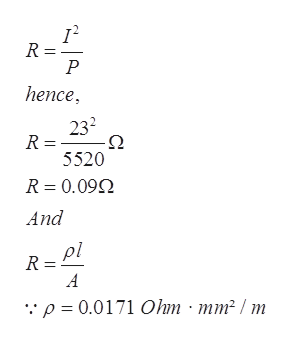# 7.If your company has just purchased a new machine which has a nameplate rating of 23 Amps at240 volts. What size conductors would you run to this machine?How many conductors would be required for this machine if it also required 120 VAC?8.

Question
1 viewshelp_outlineImage Transcriptionclose7. If your company has just purchased a new machine which has a nameplate rating of 23 Amps at 240 volts. What size conductors would you run to this machine? How many conductors would be required for this machine if it also required 120 VAC? 8. fullscreen
check_circle

Step 1

As given that machine have rating of 240 volt and 23 amp.

Therefore, power generated by machine=V×I

Power =240×23 W

Power =5520 W

Hence, Equal amount of power and current is  flowing through conductors.

Assume that the conductor material is copper

Formula for resistance ishelp_outlineImage TranscriptioncloseR = Р hence, 232 Ω R = 5520 R 0.09 And pl R A "p = 0.0171 Ohm mm2 / m fullscreen
Step 2

Assume length of wire is 1000 mm

Therefore, plugging...

### Want to see the full answer?

See Solution

#### Want to see this answer and more?

Solutions are written by subject experts who are available 24/7. Questions are typically answered within 1 hour.*

See Solution
*Response times may vary by subject and question.
Tagged in

### Electrical Engineering